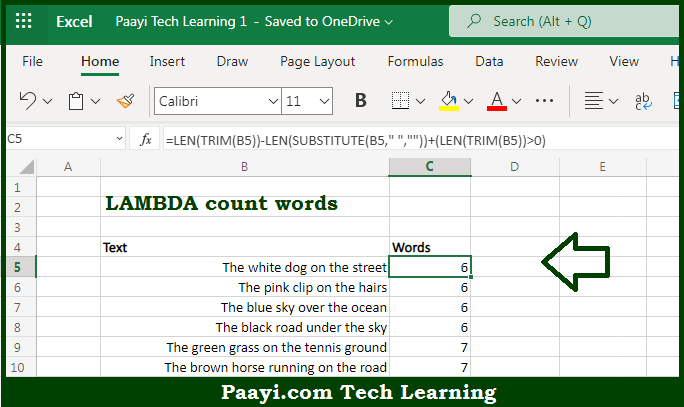# Learn How to LAMBDA Count Words in Microsoft Excel

Written by | 0 Comments | 302 Views

In this article, you will learn how to evaluate things in Dynamic Arrays with formulas in Microsoft Excel using a single/combination(s) of functions. You will also know How to LAMBDA Count Words and see the generic formula.

Learn How to LAMBDA Count Words in Microsoft Excel

The main purpose of this formula is to create a custom function to count total words in a cell. Here we will learn how to LAMBDA count words in the given data range in the workbook in Microsoft Excel. That implies, with the help of a formula based on the LAMBDA function you can able to create a custom function to count total words in a cell. So, with the help of this formula, you can able to LAMBDA count words in the given data range in the workbook in Microsoft Excel.

General Formula to LAMBDA Count Words

=LAMBDA(text,LEN(TRIM(text))-LEN(SUBSTITUTE(text," ",""))+(LEN(TRIM(text))>0))

The Explanation for LAMBDA Count WordsSo we know that with the help of the given formula above you can able to create a custom function to count total words in a cell. Here we will learn how to LAMBDA count words in the given data range in the workbook in Microsoft Excel. As we know that Excel does not provide a formula function to append or combine ranges. So you can append ranges with Power Query. You can also use the LAMBDA function to create a custom function to combine ranges. So, with the help of this formula, you can able to create a custom function to count total words in a cell. Here we will learn how to LAMBDA count words in the given data range in the workbook in Microsoft Excel.